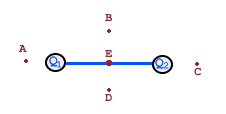# Net Electric Force

## Homework Statement[/B]
In the above figure, if Q1 and Q2 are positive, where could a negative charge be placed and have a vertically upward net force on it?

## Homework Equations

F = k|q||q| / (r^2)

## The Attempt at a Solution

I wrote the force equations for the negative charge and both positive charges, but I have no idea how to proceed from there.